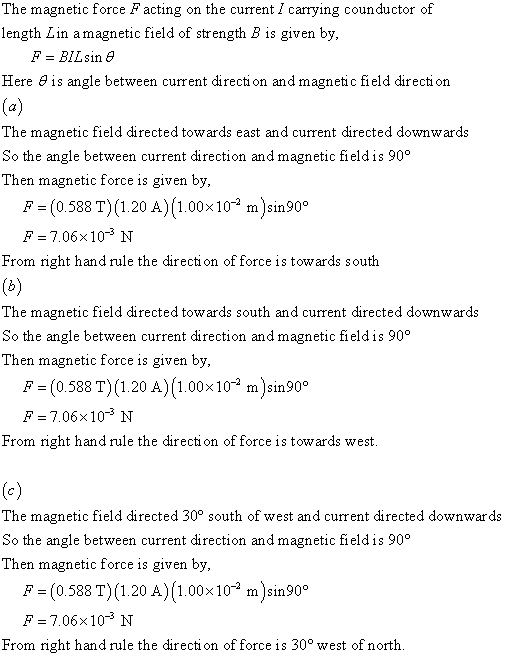#### Didn’t find what you are looking for?

Question# A straight vertical wire carries a current of 1.20 A downward in a region b/t the poles of a large superconducting electromagnet,where the magnetic fi

Other
ANSWEREDA straight vertical wire carries a current of 1.20 A downward in a region b/t the poles of a large superconducting electromagnet,where the magnetic filed has magnitude B = 0.588 T and is horizontal. What are the magnitude and direction of the magnetic force on a 1.00 cm section of the wire that is in this uniform magnetic field, if the magnetic field direction is a) east? b)south? c) 30 degrees south of west?
According to the solutions, the force stays the same for parts $$\displaystyle{a},{b},{c}:{F}={I}\cdot{l}\cdot{B}={7.06}\times{10}^{{-{3}}}$$ but for part c,why isn't the 30 degree angle used to calculate the force?...................